# CommunicatingIntent and Formal Specifications

## Marcell J. Vazquez-Chanlatte

### Qualifying Exam (March 20th, 2019)# Motivation: Robot-Human Interaction

Human-Robot interactions are becoming increasingly ubiquitous.

• Many tasks implicitly require inferring objectives of other agents.
• Success = Increased need for safety, correctness, and audit-ability.
• Can we leverage formal methods in these domains?

# Specification Mining

Exceptions concurrently developed: (Kasenberg et al CDC 2017), (Shah et al NeurIPS 2018)

# What are Demonstrations?

• A demonstration of a task is an unlabeled example where
the agent tries to perform the task (will revisit definition).
• Agency is key. Need a notion of action.

# Demonstrations - Necessary but not sufficientBut why demonstrations vs natural language, etc?

1. Natural language is ambiguous.
2. People might not mean what they say.
3. Demonstrations can be incredibly diagnostic.
4. Demonstrations can be used to teach human.# Research GoalThrough my research I seek to develop theory and tools for communicating$^*$ formal specifications.

$^*$: Terminology due to deep connection with Information Theory.

This talk will focus on learning and teaching specifications via demonstrations.

# Research Landscape and Current Contributions1. Talk focuses on (NeurIPS 2018).
Maximum Entropy IRL (Ziebart, et al. 2008) $\to$ Specification Mining.
2. Preliminary work on specification teaching and communication discusses at the end.
(CPHS 2018)
3. Occasionally you might see links which lead to longer derivations or other research/asides which can supplement the talk.

# Structure of the talk

## Act 1 - Learning Specifications

1. Formal Specification Propaganda
2. Maximum Entropy Specification Learning
(NeurIPS 2018, current work)## Act 2 - Teaching Specifications

1. Episodic Specification Teaching
(CPHS 2018, future work)
2. Cooperative Planning
future work
3. Legibility
future work# Simplifying Assumptions

1. Above domains are currently aspirations.
2. 90% of this talk we will focus on episodic learning (and teaching) with a single agent.3. Final 10% will treat inter-episodic learning (and teaching) with multiple-agents.

# Running Example

## High Level Motion Planner

1. Dynamics: Can move North, South, East, and West, but may probabilistically slip or be pushed by wind.
2. States: In a addition to position, four types of states: lava, water, drying, and recharge.
3. The dynamics are independent of the state type.
4. Example Task: Eventually recharge while avoiding Lava. If agent enters water, the agent must dry off before recharging.

# Structure of the talk

## Act 1 - Learning Specifications

1. Formal Specification Propaganda
2. Maximum Entropy Specification Learning
(NeurIPS 2018, current work)## Act 2 - Teaching Specifications

1. Episodic Specification Teaching
(CPHS 2018, future work)
2. Cooperative Planning
future work# Basic definitions

1. Assume some fixed set $S$, called states.2. A trace, $\xi$, is a sequence of states.
3. A specification(trace property), $\varphi$, is a set of traces.
4. We say $\xi$ satisfies $\varphi$, written $\xi \models \varphi$, if $\xi \in \varphi$.# Communicating Intent and Formal Specifications

1. Specification: What qualifies as acceptable behavior?
2. Derived from Formal Logic, Automata, Rewards ($\epsilon$-"optimal").3. No a-priori ordering between acceptable behaviors.4. Key question: How to compose specifications.
$\circ :$ Specification $\times$ Specification $\to$ Specification

# Communicating Intent and Formal Specifications

Key question: How to compose specifications.
$\circ :$ Specification $\times$ Specification $\to$ Specification

1. Build up complex specifications from simpler ones.
2. Can always compose as concrete the sets:3. Preferable to compose at abstract level: $(\neg a \wedge b)$4. Question: How can one compose rewards?

# Utility Maximizing AgentsAssume agent is acting in a Markov Decision process and optimizing the sum of an unknown state reward, $r(s)$, i.e,: $\begin{split} &\pi : S \times A \to [0, 1]\\ \max_{\pi} &\Big(\mathbb{E}_{s_{1:T}}\big(\sum_{i=1}^T r(s_i)~|~\pi\big)\Big) \end{split}$

1. Agent model reinforcement learning (and optimal control)
(Witten 1977, Sutton and Barto 1981)(Bellman 1957).
2. Implicit specification: Pick a trace with (approximately) optimal reward.# Inverse Reinforcement LearningAssume agent is acting in a Markov Decision process and optimizing the sum of an unknown state reward, $r(s)$, i.e,: $\max_{\pi} \Big(\mathbb{E}_{s_{1:T}}\big(\sum_{i=1}^T r(s_i)~|~\pi\big)\Big)$ Given a series of demonstrations, what reward, $r(s)$, best explains the behavior? (Abbeel and Ng 2004)

Will revisit solution techniques.

# Problems with rewards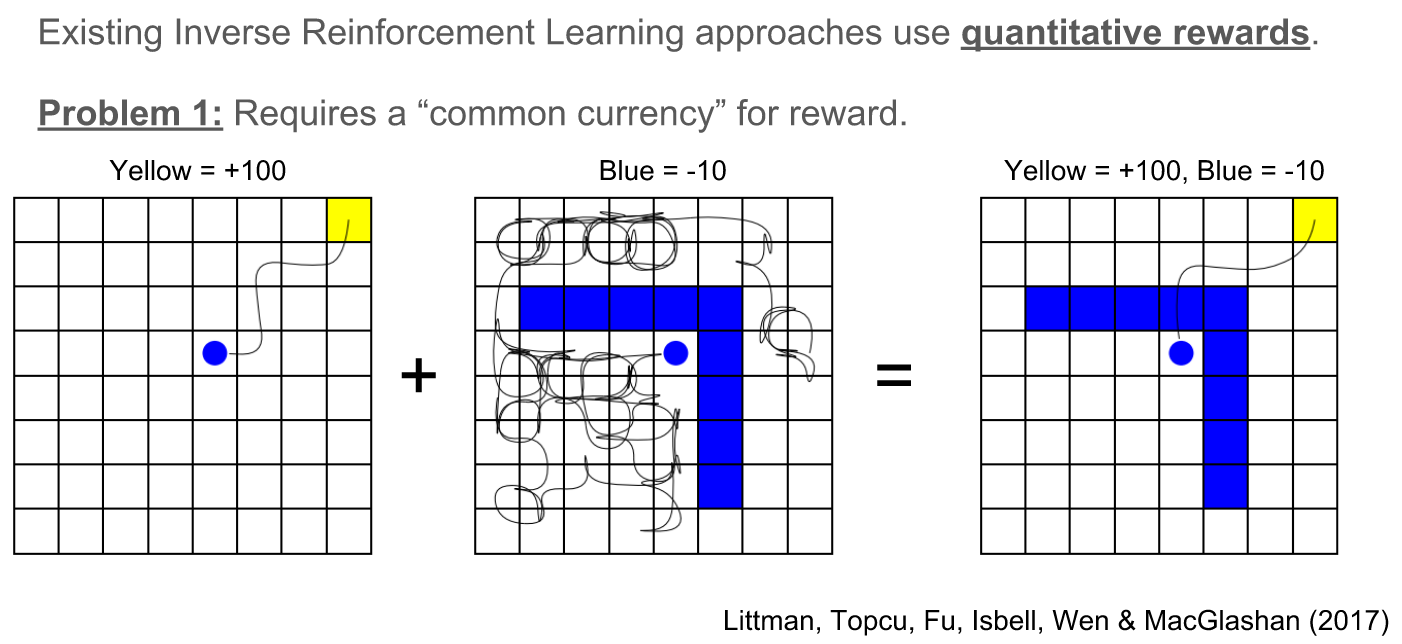How to safely compose in a dynamics invariant way?

# Problems with rewards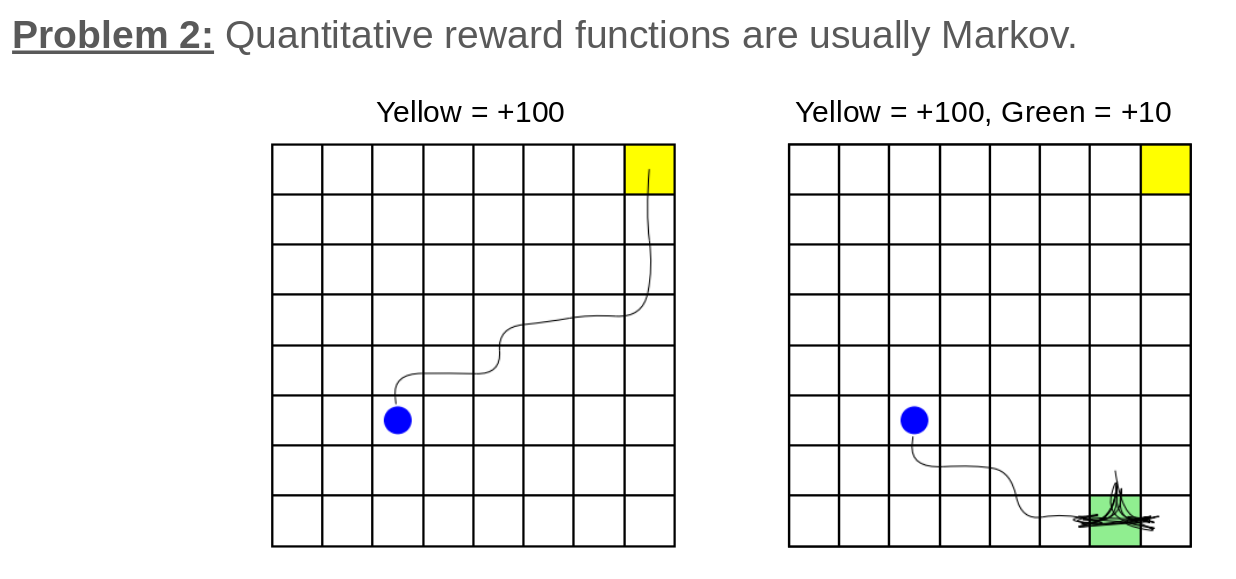1. Dynamic States != Reward States
2. Beware the curse of history (Pineau et al 2003).
Adding history can result in exponential state space explosion.

# Running Example is Compositional and non-Markovian

$\varphi = \varphi_1 \wedge \varphi_2 \wedge \varphi_3$

1. $\varphi_1$ = Eventually recharge.
2. $\varphi_2$ = Avoid lava.
3. $\varphi_3$ = If agent enters water, the agent must dry off before recharging.

# Structure of the talk

## Act 1 - Learning Specifications

1. Formal Specification Propaganda
2. Maximum Entropy Specification Learning
(NeurIPS 2018, current work)## Act 2 - Teaching Specifications

1. Episodic Specification Teaching
(CPHS 2018, future work)
2. Cooperative Planning
future work# Learning Trace Properties from Demonstrations

• A demonstration of a task $\varphi$ is an unlabeled example where
the agent tries to satisfy $\varphi$.
• Agency is key. Need a notion of action.
• Success probabilities induce an ordering.# Informal Problem Statement• Assume an agent is operating in a Markov Decision Process while trying to satisfy some unknown specification.
• Given a sequence of demonstrations, $\text{demos}$, and a collection of specifications, $\Phi$, find the specification $\varphi \in \Phi$ that best explains the agent's behavior.

Idea: Apply analog to Inverse Reinforcement Learning.

# Inverse Reinforcement LearningAssume agent is acting in a Markov Decision process and optimizing the sum of an unknown state reward, $r(s)$, i.e,: $\max_{\pi} \Big(\mathbb{E}_{s_{1:T}}\big(\sum_{i=1}^T r(s_i)~|~\pi\big)\Big)$ Given a series of demonstrations, what reward, $r(s)$, best explains the behavior? (Abbeel and Ng 2004)

1. Note: There is no unique solution as posed! $\Pr(\xi~|~\text{demos}, r) = ?$
2. Idea: Disambiguate via the Principle of Maximum Entropy. (Ziebart, et al. 2008)

# Principle Maximum Entropy Intuition

Key Idea: Don't commit to any particular prediction more than the data forces you too.1. Minimum Entropy Distribution: Assume agent's policy exactly matches the demonstrations.
2. Maximum Entropy Distribution: Find distribution that matches measured moments, but has no additional bias.
3. Examples:
• Uniform: [0, 1] domain and no moment matching constraints.
• Exponential: Positive reals domain and match expected value.

# Boolean Rewards Intuition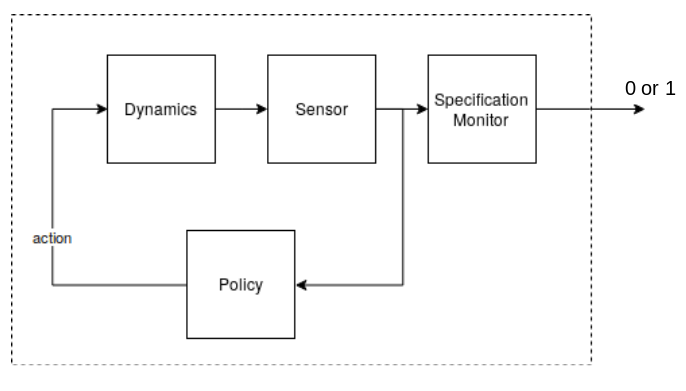# Boolean Rewards Intuition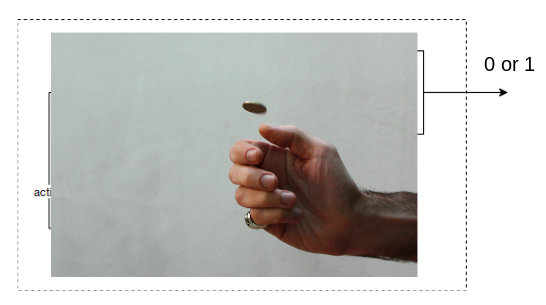Observe satisfaction probability, $p_\varphi$.

# Boolean Rewards Intuition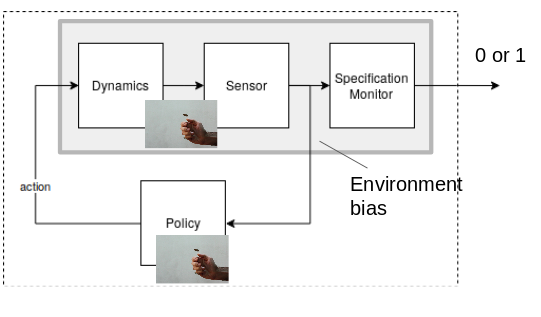Satisfaction probability, $p_\varphi$, affected by policy and how "easy" the specification/dynamics combination is.# Boolean Rewards Max Ent Distribution$\begin{split} &p_\varphi \triangleq \Pr(\xi \models \varphi~|~\text{teacher policy})\\ &q_\varphi \triangleq \Pr(\xi \models \varphi ~|~\text{random actions}) \end{split}$
1. The Maximum Entropy Distribution given $p_\varphi$ is: $\Pr(s_{1:T} ~|~\text{demos}, \varphi) \propto \begin{cases} \frac{p_\varphi}{q_\varphi}& \text{if } s_{1:T} \in \varphi\\ \frac{p_{\neg \varphi}}{q_{\neg \varphi}}& \text{if } s_{1:T} \notin \varphi \end{cases}$
2. Note: When the dynamics are deterministic, this recovers the size principle from concept learning! (Tenenbaum 1999)

# Maximum Entropy Likelihood given i.i.d. demos

$\Pr(s_{1:T} ~|~\text{demos}, \varphi) \propto \begin{cases} \frac{p_\varphi}{q_\varphi}& \text{if } s_{1:T} \in \varphi\\ \frac{p_{\neg \varphi}}{q_{\neg \varphi}}& \text{if } s_{1:T} \notin \varphi \end{cases}$

• Teacher at least as good as random: $p_\varphi \geq q_\varphi$
• Demonstrations, $\text{demos} = \{\xi_1, \xi_2, \xi_3, \ldots \xi_n \}$, given i.i.d.
• Demonstrations are representative: $p_\varphi \approx \frac{|\text{demos}~\cap~\varphi|}{n}$
• $P_\varphi \triangleq \text{coin with bias } p_\varphi ~~~~~Q_\varphi \triangleq \text{coin with bias } q_\varphi$

... Algebra ...

$\Pr(\text{demos}~|~ \varphi) \propto 1[p_\varphi \geq q_\varphi]\exp{\Big(n\cdot D_{KL}(P_\varphi || Q_\varphi)\Big )}$

Aside: Can be interpreted as quantifying the atypicality of $\text{demos}$ over random action hypothesis.
(Sanov's Theorem 1957)

# Model Summary$P_\varphi \triangleq \text{coin with bias } p_\varphi ~~~~~Q_\varphi \triangleq \text{coin with bias } q_\varphi$

$\Pr(\text{demos}~|~ \varphi) \propto 1[p_\varphi \geq q_\varphi]\exp{\Big(n\cdot D_{KL}(P_\varphi || Q_\varphi)\Big )}$

# How to optimize?

$\Pr(\text{demos}~|~\varphi) \propto 1[p_\varphi \geq q_\varphi]\exp{\Big(n \cdot D_{KL}(P_\varphi || Q_\varphi)\Big )}$
1. Suppose robot has a concept class, $\Phi$, of specifications.
2. Want to make $p_\varphi$ and $q_\varphi$ as separate as possible.3. Gradients are not well defined: $p_{\varphi}$ and $q_{\varphi}$ change discretely.

Proposition 1:

$\varphi \subseteq \varphi' \implies \Big (p_{\varphi} \leq p_{\varphi}' \text{ and } q_{\varphi} \leq q_{\varphi}' \Big).$# Monotonicity of Maximum Entropy Model

$\Pr(\text{demos}~|~\varphi) \propto 1[p_\varphi \geq q_\varphi]\exp{\Big(n\cdot D_{KL}(P_\varphi || Q_\varphi)\Big )}$

Proposition 2:

The maximum entropy model above monotonically decreases in $q_\varphi$.

$f_i(x) \triangleq \Pr\left(\text{demos}~|~\varphi, p_\varphi = \frac{i}{n}, q_\varphi=x\right)$

# Monotonicity of Maximum Entropy Model

Proposition 2:

The maximum entropy model above monotonically decreases in $q_\varphi$.

$f_i(x) \triangleq \Pr\left(\text{demos}~|~\varphi, p_\varphi = \frac{i}{n}, q_\varphi=x\right)$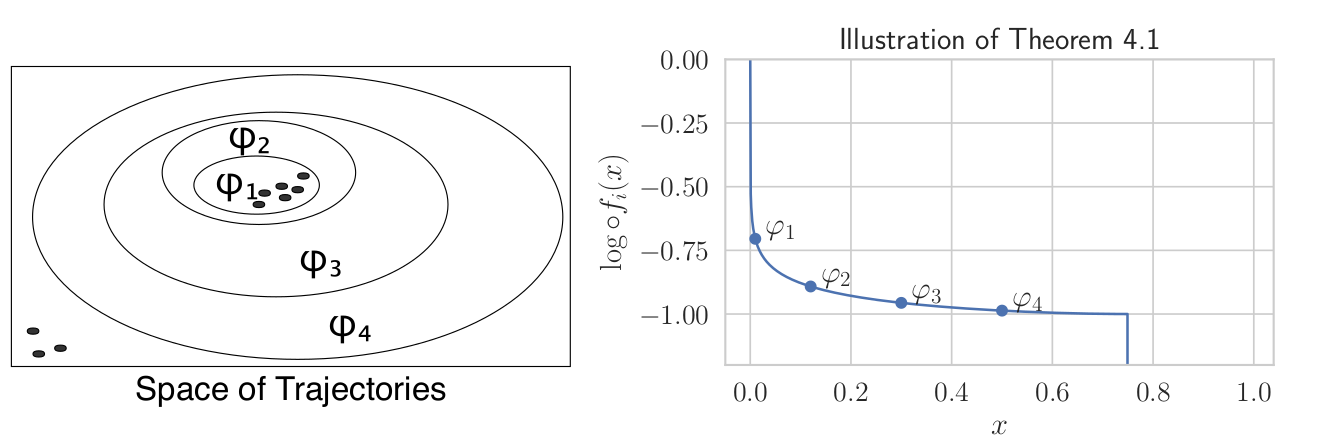# Caution - Can overfit• Q: What is the likelihood of generating $\text{demos}$ given $\varphi = \text{demos}$?
• Mitigations:
1. Constrain concept class, $\Phi \subsetneq S^\omega$.
Examples: simple temporal logic formula, number of states in the automata, circuit complexity, etc.
2. Carefully construct priors.
Examples: exponential in description length, entropy, or circuit complexity.
3. Avoid Maximum a Posteriori Estimation by marginalizing over the whole distribution.
(reduction to $L^*$).

# Structure of the talk

## Act 1 - Learning Specifications

1. Formal Specification Propaganda
2. Maximum Entropy Specification Learning
(NeurIPS 2018, current work)## Act 2 - Teaching Specifications

1. Episodic Specification Teaching
(CPHS 2018, future work)
2. Cooperative Planning
future work# Human Understandable $\neq$ Machine Understandable1. Co-Op IRL / Showing vs Doing:
Teacher provides demonstrations that disambiguate objective (Hadfield-Menell et al 2016) (Ho et al 2016).2. Idea: Can these differences be explained by optimizing for our MaxEnt Specification Learner?

# Teaching Problem Statement1. Assume the human performs Maximum Likelihood Estimation over a concept class $\Phi$ using a likelihood compatible with: $\Pr(\text{demos}~|~\varphi) \propto 1[p_\varphi \geq q_\varphi]\exp{\Big(n\cdot D_{KL}(P_\varphi || Q_\varphi)\Big )}.$
2. For a fixed $n \in \mathbb{N}$, find $n$ demonstrations that maximally disambiguate $\varphi^*$ from all other specifications in $\Phi$: $\max_{\text{demos}}\min_{\substack{\varphi \in \Phi\\\varphi \neq \varphi^*}}\Big(\Pr(\text{demos}~|~\varphi^*) - \Pr(\text{demos}~|~\varphi)\Big).$

# Select Demonstrations and World to Maximally Disambiguate

$\Pr(\text{demos}~|~\varphi) \propto 1[p_\varphi \geq q_\varphi]\exp{\Big(n\cdot D_{KL}(P_\varphi || Q_\varphi)\Big )}$
• Can control $p$ by changing the demonstrations.
• Can control $q$ by changing the world, e.g, the starting position.# Induced OrderingConcept Class: $\Phi = \{\varphi^*, \varphi', \varphi''\}$

$\varphi^* = \text{Eventually Recharge}$
$\varphi' = \text{Avoid Lava}$
$\varphi'' = \varphi^* \wedge \varphi'$

# Structure of the talk

## Act 1 - Learning Specifications

1. Formal Specification Propaganda
2. Maximum Entropy Specification Learning
(NeurIPS 2018, current work)## Act 2 - Teaching Specifications

1. Episodic Specification Teaching
(CPHS 2018, future work)
2. Cooperative Planning
future work# Communicating Trace Properties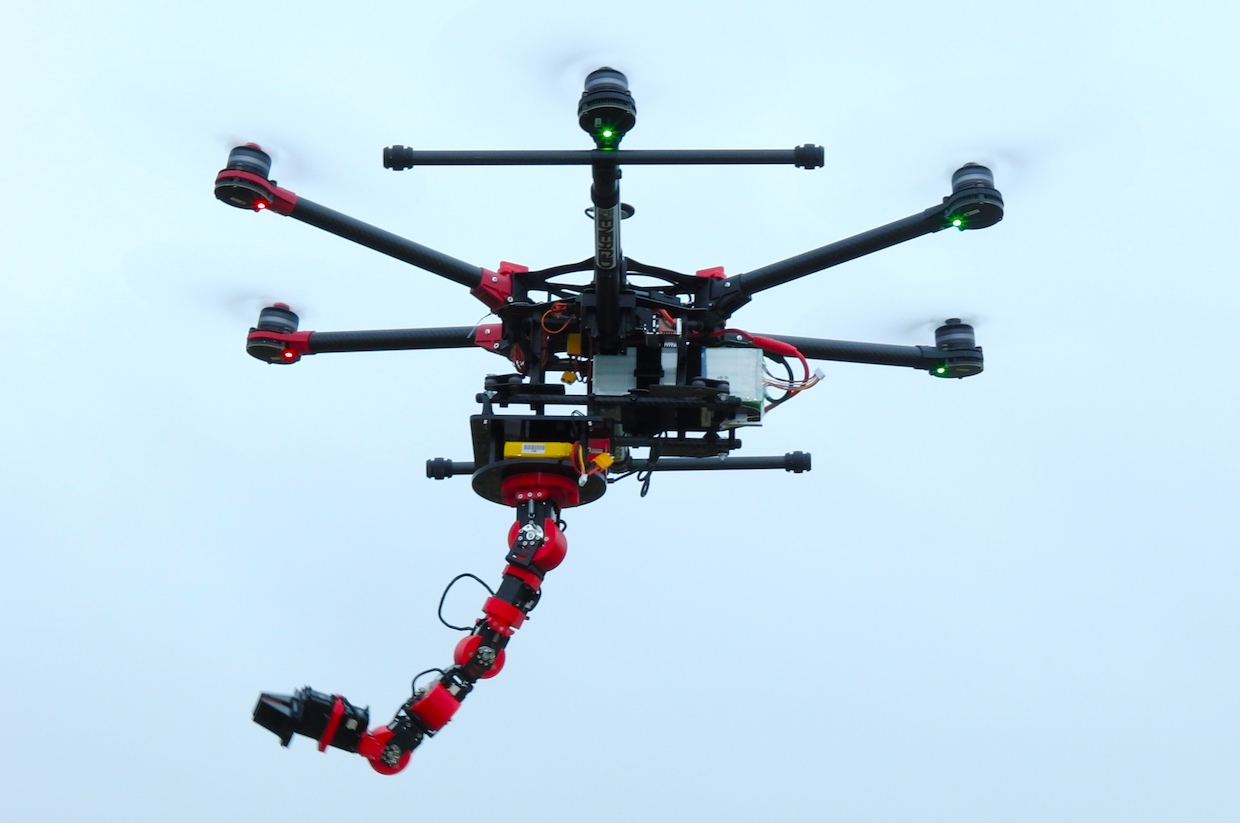Drone with Human Operated ManipulatorPhoto: Energid1. Proposal: Receding Horizon Learning and Teaching for collaborative control.
• Infer objective, $\varphi_G$, of other agent based on their past actions.
• Objective 1 - Cooperation: Plan trajectory that helps the agent satisfy $\varphi_G$ (inspired by Liu et al., AAMAS 2016).
• Objective 2 - Information Gathering: Disambiguate other agent's objective. (inspired by Sadigh et al. IROS 2016)
• Apply first action of trajectory and replan.

A complete solution should also factor trajectory Legibility (Dragan et al 2013)

# Act 3

Challenge Problems

# Challenge Problems

1.How to quickly communicate to new operatorfeatures and constraints (ODDs) of new vehicle, e.g, Tesla Autopilot.

# Fin# Exploiting Binary Rewards# Exploiting Binary Rewards# Exploiting Binary Rewards# Exploiting Binary Rewards# Binary Decision DiagramsObservation: Early "obviously correct" moves preferred.

# CommunicatingIntent and Formal Specifications

1. High level intent are specifications.
2. Two types of intent: High level objective vs low level planning.
3. Low level planning requires a dynamics model.
4. Did the agent intend to enter the lava?5. Success probabilities induce an ordering.# Maximum Entropy Inverse Reinforcement Learning$\Pr(o_i = 1~|~s_i, a_i, r) \propto e^{r(s_i)}$

1. Idea: Disambiguate via the Principle of Maximum Entropy. (Ziebart, et al. 2008)(Cover and Thomas 2012)
2. Can be derived using inference on above Graphical Model. (Levine 2018)
3. Resulting distribution: $\Pr(s_{1:T}, a_{1:T}~|~o_{1:T}=1, r) \propto \Big( \prod_{i=1}^{T-1} \Pr(s_{i+1}~|~s_i, a_i)\Big) \Big( e^{\sum_{i=1}^T r(s_i, a_i))}\Big)$
4. Tune $r$ to match data, e.g, first moment of features.

# Trace properties as Boolean Rewards

1. Assume episode length $T \in \mathbb{N}$.
2. Consider $\varphi$ decidable by time, $T \in \mathbb{N}$, e.g, $\varphi \subseteq S^T$.
3. Define episode reward via $r_\varphi(s_{1:T}) = \mathbf{1}[s_{1:T} \in \varphi]$.
4. Note: Made Markovian by unrolling Markov Decision Process.
5. Example: $\varphi(\xi) =$"Visit blue by t=2".# Trace properties as Boolean Rewards1. Maximum Entropy Distribution:

$\Pr(s_{1:T}, a_{1:T}~|~o_{1:T}=1, r) \propto \Big( \prod_{i=1}^{T-1} \Pr(s_{i+1}~|~s_i, a_i)\Big) \Big( e^{\sum_{i=1}^T r(s_i, a_i))}\Big)$

2. Maximum Entropy Distribution becomes:

$\Pr(s_{1:T}, a_{1:T}~|~o_{1:T}=1, \varphi) \propto e^{\lambda\cdot r_\varphi} \prod_{i=1}^{T-1} \Pr(s_{i+1}~|~s_i, a_i)$

where $\lambda$ is tuned to match $\mathbb{E}_{s_{1:T}}(r_\varphi(s_{1:T})~|~\text{demos})$.

Note: Boolean rewards have only two values.

# Bounded Trace properties as Boolean Rewards1. Maximum Entropy Distribution:

$\Pr(s_{1:T}, a_{1:T}~|~o_{1:T}, \varphi) \propto e^{\lambda\cdot r_\varphi} \prod_{i=1}^{T-1} \Pr(s_{i+1}~|~s_i, a_i)$

2. $W_\varphi \triangleq \sum_{s_{1:T} \in \varphi}\prod_{i=1}^T(\Pr(s_{i+1}~|~s_i, a_i))$.
3. Two constraints:

$\begin{split} \text{Probabilities sum to 1: } &~~~~Z_\varphi = W_{\neg \varphi} e^0 + W_{\varphi} e^\lambda\\ \text{Feature Matching: }&~~~~Z_\varphi \mathbb{E}_{s_{1:T}}(r_\varphi(s_{1:T}~|~\text{demos})) = 1\cdot e^{\lambda}W_\varphi + 0\cdot e^{0} W_\varphi = e^{\lambda}W_\varphi \end{split}$

# Bounded Trace properties as Boolean Rewards

$\begin{split} &Z_\varphi = W_{\neg \varphi} e^0 + W_{\varphi} e^\lambda\\ &Z_\varphi \mathbb{E}_{s_{1:T}}(r_\varphi(s_{1:T})~|~\text{demos}) = e^{\lambda 1}W_\varphi\\\\ &p_\varphi \triangleq \mathbb{E}_{s_{1:T}}(r_\varphi(s_{1:T})~|~\text{demos})\\ &q_\varphi \triangleq \mathbb{E}_{s_{1:T}}(r_\varphi(s_{1:T})~|~\text{random actions}) = W_\varphi / Z_\varphi \end{split}$

.... Algebra ...

$\Pr(s_{1:T}~|~\text{demos}, \varphi) \propto \begin{cases} \frac{p_\varphi}{q_\varphi}& \text{if } s_{1:T} \in \varphi\\ \frac{p_{\neg \varphi}}{q_{\neg \varphi}}& \text{if } s_{1:T} \notin \varphi \end{cases}$

# Act 1.8

## Post NeurIPS 2018

1. Maximum A Posteriori $\to L^*$ Automata Learning
2. Maximum Entropy $\to$ Maximum Causal Entropy

# MAP considered Harmful

1. Maximum a posteriori estimation throws away all of the distribution information.# MAP considered Harmful

1. Maximum a posteriori estimation throws away all of the distribution information.2. Without proper priors this can lead to overfitting.
3. Note: Marginalizing results in a "soft-decision": $\Pr(\xi \in \varphi_*~|~\text{demos}) \propto \int_{\substack{\varphi \in \Phi\\\varphi\ni \xi}} \Pr(\xi~|~\text{demos}, \varphi)\Pr(\varphi).$
4. Set of $\kappa$-"probable" traces is a trace property. $\varphi_\kappa \triangleq \Big\{\xi \in S^* ~.~\Pr(\xi \in \varphi_*~|~\text{demos}) > \kappa \Pr(\xi \notin \varphi_*~|~\text{demos})\Big\}$
where $\kappa \geq 1$.

# Angluin Style Learning ($L^*$)

Learning Regular Languages (as DFAs) with minimally adequate teacher is polytime in the number of states. (Angluin 1987)1. Membership$(\xi):$ Is $\xi$ a member of $\varphi_*$? (Y / N)
2. Equivalence$(\varphi)$: Is $\varphi$ equivalent to $\varphi^*$? (Y / Counter Example)

Idea: Use $\kappa$ probable decision as membership oracle.

Benefits: Avoid overfitting + Learn outside of concept class.

# Angluin Style Learning ($L^*$)

Learning Regular Languages (as DFAs) with minimally adequate teacher is polytime in the number of states. (Angluin 1987)## Proposed Teacher

1. Membership($\xi$): $\Pr(\xi \in \varphi_*~|~\text{demos}) > \kappa \Pr(\xi \notin \varphi_*~|~\text{demos})$.
2. NoisyEquivalence($\varphi$): Sample many $\xi_i$ and check if:
$\xi_i \in \varphi \iff \text{Membership}(\xi_i).$

# Maximum Entropy vs Maximum Causal Entropy• Problem: $\Pr(s_{i+1}~|~s_i, a_i, o_i=1, r) \neq \Pr(s_{i+1}~|~s_i, a_i)$.
• Consequence: Agent implicitly assumes it has some control over dynamics.
$\Pr(s_{1:T}, a_{1:T}~|~o_{1:T}=1, r) \propto \Big( \prod_{i=1}^{T-1} \Pr(s_{i+1}~|~s_i, a_i)\Big) \Big( e^{\sum_{i=1}^T r(s_i, a_i))}\Big)$
• Fix: Condition on causal information flow (Ziebart, et al. 2010, Levine 2018).
• Implication: $\pi(s_i~|a_i)$ Given via Soft Bellman Backup.
$\pi(a_i~|s_i) = \exp(Q(s_i, a_i) - V(s_i))\\ ~\\ Q(s_i, a_i) = r(s_i, a_i) + \mathbb{E}[V(s_{i+1})~|~s_i, a_i]\\ V(s_i) = \text{softMax}_{a\in A}(Q(s_i, a_i))\\$

# Combinatorial Explosion!

## Problem: How to do Bellman backup after unrolling?## Proposed Solution

1. Model Markov Decision process as a probabilistic sequential circuit (SAT 2019 in submission)
2. Represent tree via ordered Binary Decision Diagrams (Bryant 1992)Legibility: Inference along the whole trajectory should be considered. (Dragan et al 2013).

Proposal: Use Maximum Causal Entropy BDD to select most legible demonstrations.1. Note: Shorter paths in BDD correlate to faster inference along path.
2. "More obviously correct" actions have higher probability.
3. Idea: Look for path that is comparatively short in objective's bdd.

# Structure of the talk

## Act 1 - Learning Specifications

1. Formal Specification Propaganda
2. Maximum Entropy Specification Learning